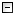Home Control Panel Login Register Search Submit Logout About me Contact meMy ResearchWeb This Site
 Home > My Research > Math and Trigonometry functions in Excel
Math and Trigonometry functions in Excel
2005-12-05    johnny       Read: 3257 times
Cite this page: johnny (2005). Math and Trigonometry functions in Excel. Retrieved June 18, 2021, from http://www.psychstat.org/us/article.php/47.htm.
 Math and Trigonometry functions in Excel ABS          Returns the absolute value of a number ACOS           Returns the arccosine of a number ACOSH                Returns the inverse hyperbolic cosine of a number ASIN                Returns the arcsine of a number ASINH                Returns the inverse hyperbolic sine of a number ATAN                Returns the arctangent of a number ATAN2                Returns the arctangent from x- and y-coordinates ATANH                Returns the inverse hyperbolic tangent of a number CEILING                Rounds a number to the nearest integer or to the nearest multiple of significance COMBIN                Returns the number of combinations for a given number of objects COS                Returns the cosine of a number COSH                Returns the hyperbolic cosine of a number COUNTIF                Counts the number of nonblank cells within a range that meet the given criteria DEGREES                Converts radians to degrees EVEN                Rounds a number up to the nearest even integer EXP                Returns e raised to the power of a given number FACT                Returns the factorial of a number FACTDOUBLE                Returns the double factorial of a number FLOOR                Rounds a number down, toward zero GCD                Returns the greatest common divisor INT                Rounds a number down to the nearest integer LCM                Returns the least common multiple LN                Returns the natural logarithm of a number LOG                Returns the logarithm of a number to a specified base LOG10                Returns the base-10 logarithm of a number MDETERM                Returns the matrix determinant of an array MINVERSE                Returns the matrix inverse of an array MMULT                Returns the matrix product of two arrays MOD                Returns the remainder from division MROUND                Returns a number rounded to the desired multiple MULTINOMIAL                Returns the multinomial of a set of numbers ODD                Rounds a number up to the nearest odd integer PI                Returns the value of pi POWER                Returns the result of a number raised to a power PRODUCT                Multiplies its arguments QUOTIENT                Returns the integer portion of a division RADIANS                Converts degrees to radians RAND                Returns a random number between 0 and 1 RANDBETWEEN                Returns a random number between the numbers you specify ROMAN                Converts an arabic numeral to roman, as text ROUND                Rounds a number to a specified number of digits ROUNDDOWN                Rounds a number down, toward zero ROUNDUP                Rounds a number up, away from zero SERIESSUM                Returns the sum of a power series based on the formula SIGN                Returns the sign of a number SIN                Returns the sine of the given angle SINH                Returns the hyperbolic sine of a number SQRT                Returns a positive square root SQRTPI                Returns the square root of (number * pi) SUBTOTAL                Returns a subtotal in a list or database SUM                Adds its arguments SUMIF                Adds the cells specified by a given criteria SUMPRODUCT                Returns the sum of the products of corresponding array components SUMSQ                Returns the sum of the squares of the arguments SUMX2MY2                Returns the sum of the difference of squares of corresponding values in two arrays SUMX2PY2                Returns the sum of the sum of squares of corresponding values in two arrays SUMXMY2                Returns the sum of squares of differences of corresponding values in two arrays TAN                Returns the tangent of a number TANH                Returns the hyperbolic tangent of a number TRUNC                Truncates a number to an integer Submitted by: johnny
 Add a comment View comment Add to my favorite Email to a friend Print
If you want to rate, please login first, or click here to register. Or you can use USERNAME: guest and PASSWORD: guest to log in.
Average score 0, based on 0 comments1 2 3 4 5 6 7 8 9 10
 Copyright © 2003-13 Zhiyong Zhang \'s Psychometric WebsiteMaintained by Zhiyong Zhang